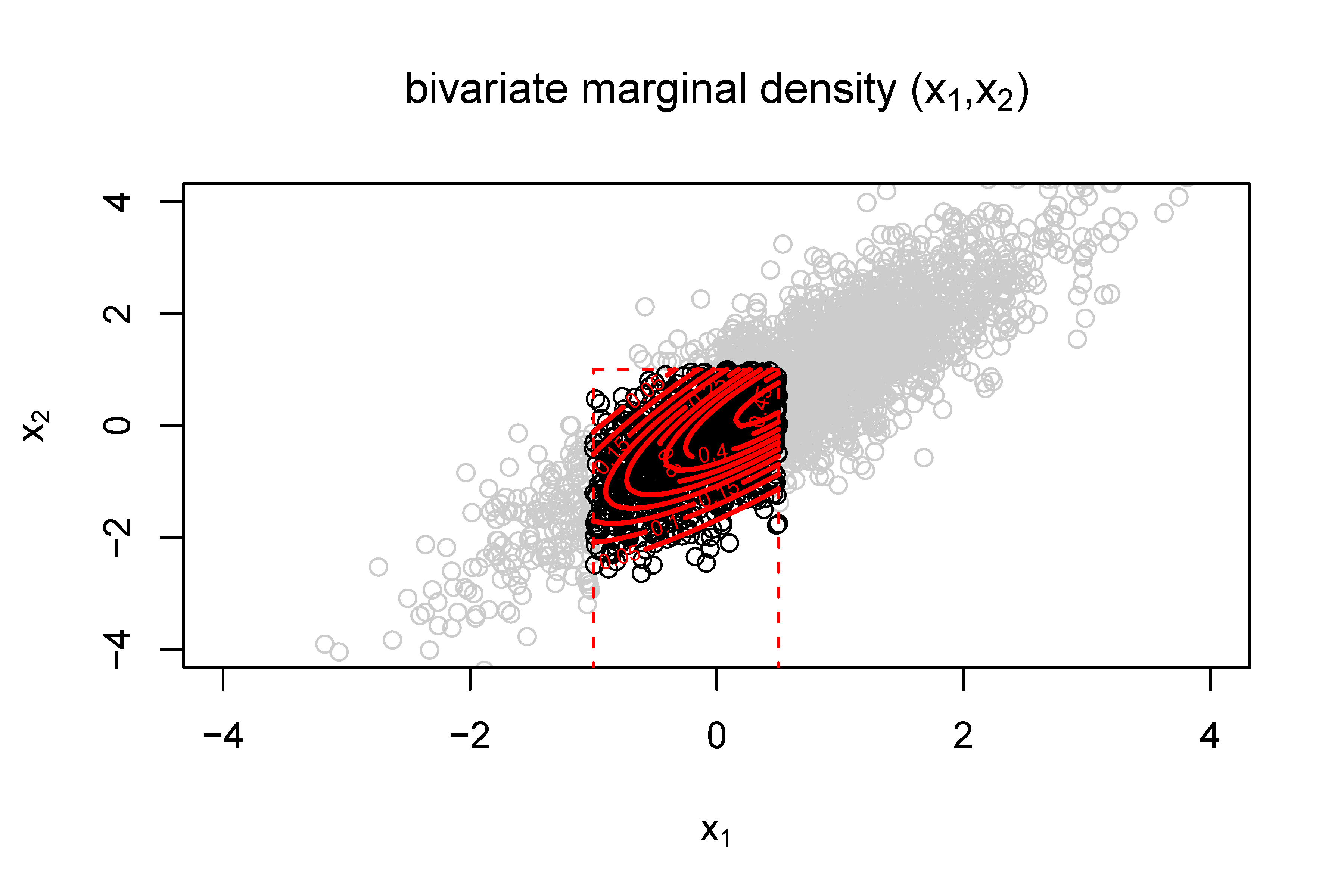# Moments Calculation for the Doubly Truncated Multivariate Normal Density

## Authors

• B. G. Manjunath School of Mathematics and Statistics, University of Hyderabad
• Stefan Wilhelm Department of Finance, University of Basel

## Keywords:

Multivariate normal, Double truncation, Moment generating function, Bivariate marginal density function, Graphical models, Conditional independence

## Abstract

In the present article, we derive an explicit expression for the truncated mean and variance for the multivariate normal distribution with arbitrary rectangular double truncation. We use the moment generating approach of Tallis (1961) and extend it to general μ, Σ and all combinations of truncation. As part of the solution, we also give a formula for the bivariate marginal density of truncated multinormal variates. We also prove an invariance property of some elements of the inverse covariance after truncation. Computer algorithms for computing the truncated mean, variance and the bivariate marginal probabilities for doubly truncated multivariate normal variates have been written in R and are presented along with three examples.2021-05-30

## How to Cite

Manjunath, B. G., & Wilhelm, S. (2021). Moments Calculation for the Doubly Truncated Multivariate Normal Density. Journal of Behavioral Data Science, 1(1), 17–33. https://doi.org/10.35566/jbds/v1n1/p2

## Section

Theory and Methods